# Rate Analysis for Cement Mortar -Calculate Quantity and CostReading time: 1 minute

Rate analysis for cement mortar requires estimation of materials for cement mortar, i.e. quantity of cement and sand required for 1m3 for various proportions, i.e., CM 1:2, 1:4, 1:6, 1:8 etc. So, let us first calculate quantity of cement and sand required for 1m3 of cement mortar. For 1m3 of wet cement mortar, 1.3m3 of cement and sand is required, due to presence of voids in sand in dry state. So, cement and sand quantity is calculated for 1.3m3 of cement mortar.

## Estimation of Materials for Cement Mortar:

Steps for calculation of quantity of cement and sand for cement mortar:Consider, we want to calculate quantity of cement and sand for CM 1:X, where, 1 is proportion of cement and X is proportion of sand. Then, quantity of cement shall be calculated as: Quantity of cement =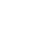Above formula gives quantity in volume. To calculate the quantity of cement in number of bags, we have to divide it by volume of cement of one bags. The volume of one bag of cement is 0.0347 m3. Therefore, number of bags of cement =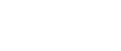Quantity of sand =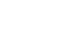For example, for a proportion of CM 1:4, Quantity of cement in bags =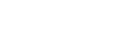=7.492 bags Quantity of sand =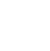= 1.04 m3 of sand.

## Estimation of Labour and Tools for cement mortar:

For 1m3 of cement mortar, semi-skilled labour is required for 0.27 days for mixing, unskilled labour for 0.26 for carrying of cement, sand and water. Hire charges for mechanical mixer is taken in lumpsum of can be taken as 0.27 days for mixing 1cum of mortar.

## Rate Analysis of cement mortar:

Rate analysis of cement mortar requires the rate of cement and sand multiplied with their respective quantity. Cost of labour and tools and tackles for the cement mortar is also calculated. Cost of cement, sand, labour and tools depend on local availability and local rates. When, quantity of cement, sand, labour and tools are multiplied by its rates, and a contractors profit of 10-20% is assumed, the total sum of the amount is taken as the cost of 1m3 of cement mortar, which is shown in the file below. Download: Rate Analysis for Cement Mortar Read More: Rate Analysis of Civil Works -Elements and Requirements Rate Analysis of Plastering with Cement Mortar -Quantity Calculation Quantity of Cement & Sand Calculation in Mortar Analysis of Rates for Civil Engineering Works Rate Analysis for Brick Masonry Quantity & Rate Analysis for Reinforced Concrete